# SARIMA vs Prophet: Forecasting Seasonal Weather Data

## ARIMA and Prophet are major time series tools used to forecast future values.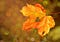Source: Photo by castleguard from Pixabay

For this particular example, a monthly weather dataset from 1941 for Dublin Airport, Ireland from the Irish weather broadcaster Met Éireann is used, and an ARIMA model is constructed to forecast mean temperature readings using R. A Prophet model is then constructed in Python to conduct forecasts on the same set of data.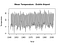Source: R Output

# Part 1: SARIMA

The purpose of ARIMA is to determine the nature of the relationship between our residuals, which would provide our model with a certain degree of forecasting power.

An ARIMA model consists of coordinates (p, d, q):

• p stands for the number of autoregressive terms, i.e. the number of observations from past time values used to forecast future values. e.g. if the value of p is 2, then this means that two previous time observations in the series are being used to forecast the future trend.
• d denotes the number of differences needed to make the time series stationary (i.e. one with a constant mean, variance, and autocorrelation). For instance, if d = 1, then it means that a first-difference of the series must be obtained to transform it into a stationary one.
• q represents the moving average of the previous forecast errors in our model, or the lagged values of the error term. As an example, if q has a value of 1, then this means that we have one lagged value of the error term in the model.

However, SARIMA is so-called since the S stands for the seasonal component in the series.

The time series is firstly defined in R as follows:

`> meant <- ts(mydata\$meant[1:732], start = c(1941,11), frequency = 12)> plot(meant,type="l",ylab="Temperature")> title("Mean Temperature - Dublin Airport")`

Seasonality is a significant concern when it comes to modelling time series. Seasonality is a particularly endemic feature of weather data — hence why many parts of the world have four seasons!

When seasonality is not accounted for, one risks erroneous forecasts of the data. While one could forecast a mean value for a particular time series, the peaks and valleys around that mean affect the forecasts for that time series significantly.

In this regard, ARIMA needs to be modified in order to include a seasonal component.

`ARIMA(p, d, q) × (P, D, Q)S`

with p = non-seasonal AR order, d = non-seasonal differencing, q = non-seasonal MA order, P = seasonal AR order, D = seasonal differencing, Q = seasonal MA order, and S = time span of repeating seasonal pattern.

While the auto.arima function in R will select the best p, d, q coordinates automatically based on the model with the lowest BIC (Bayesian Information Criterion), it is always a good idea to manually analyse the time series in the first instance to ensure that the configuration makes sense.

Here are the ACF and PACF plots:

ACF

In this instance, we can see that the strongest positive correlation comes at lag 12, which arises after a period of negatively correlated lags (4 to 8). This is expected since the temperatures during this period would be markedly different to that at lag 0.

In this regard, 12 is the appropriate seasonal parameter for the model.

PACF

For the partial autocorrelation plot, we see that there is a strong cutoff in the correlation at lag 1. This implies that the series follows an AR(1) process and the appropriate value for p=1.

Now, the time series is defined and the components are analysed:

From the above, we see that there is a clear seasonal component present in the time series. As also indicated by the ACF plot, the ARIMA model will need a seasonal component attached.

Using the aforementioned data, the following procedures are carried out in R:

• auto.arima is used to examine the best ARIMA configuration for the training data (the first 80% of all temperature data).
• The predicted values are then compared to the test values (the latter 20% of the data) to determine the model accuracy.
• Finally, the Ljung-Box test is used to determine if the data is independently distributed or exhibits serial correlation.

To determine the ARIMA configuration, the auto.arima function in R is used.

`> # ARIMA> fitlnweather<-auto.arima(meant, trace=TRUE, test="kpss", ic="bic")Fitting models using approximations to speed things up... ARIMA(2,0,2)(1,1,1) with drift         : Inf ARIMA(0,0,0)(0,1,0) with drift         : 2700.554 ARIMA(1,0,0)(1,1,0) with drift         : 2489.563 ARIMA(0,0,1)(0,1,1) with drift         : Inf ARIMA(0,0,0)(0,1,0)                    : 2693.995 ARIMA(1,0,0)(0,1,0) with drift         : 2681.764 ARIMA(1,0,0)(2,1,0) with drift         : 2419.911 ARIMA(1,0,0)(2,1,1) with drift         : 2311.394 ARIMA(1,0,0)(1,1,1) with drift         : 2288.846 ARIMA(1,0,0)(0,1,1) with drift         : Inf ARIMA(1,0,0)(1,1,2) with drift         : Inf ARIMA(1,0,0)(0,1,2) with drift         : Inf ARIMA(1,0,0)(2,1,2) with drift         : Inf ARIMA(0,0,0)(1,1,1) with drift         : 2330.622 ARIMA(2,0,0)(1,1,1) with drift         : Inf ARIMA(1,0,1)(1,1,1) with drift         : 2294.973 ARIMA(0,0,1)(1,1,1) with drift         : 2307.532 ARIMA(2,0,1)(1,1,1) with drift         : Inf ARIMA(1,0,0)(1,1,1)                    : 2282.273 ARIMA(1,0,0)(0,1,1)                    : Inf ARIMA(1,0,0)(1,1,0)                    : 2482.985 ARIMA(1,0,0)(2,1,1)                    : 2305.159 ARIMA(1,0,0)(1,1,2)                    : Inf ARIMA(1,0,0)(0,1,0)                    : 2675.208 ARIMA(1,0,0)(0,1,2)                    : Inf ARIMA(1,0,0)(2,1,0)                    : 2413.332 ARIMA(1,0,0)(2,1,2)                    : Inf ARIMA(0,0,0)(1,1,1)                    : 2324.069 ARIMA(2,0,0)(1,1,1)                    : Inf ARIMA(1,0,1)(1,1,1)                    : 2288.405 ARIMA(0,0,1)(1,1,1)                    : 2300.974 ARIMA(2,0,1)(1,1,1)                    : InfNow re-fitting the best model(s) without approximations... ARIMA(1,0,0)(1,1,1)                    : Inf ARIMA(1,0,1)(1,1,1)                    : Inf ARIMA(1,0,0)(1,1,1) with drift         : Inf ARIMA(1,0,1)(1,1,1) with drift         : Inf ARIMA(0,0,1)(1,1,1)                    : Inf ARIMA(1,0,0)(2,1,1)                    : Inf ARIMA(0,0,1)(1,1,1) with drift         : Inf ARIMA(1,0,0)(2,1,1) with drift         : Inf ARIMA(0,0,0)(1,1,1)                    : Inf ARIMA(0,0,0)(1,1,1) with drift         : Inf ARIMA(1,0,0)(2,1,0)                    : 2423.105Best model: ARIMA(1,0,0)(2,1,0)> fitweatherarimaSeries: meant ARIMA(1,0,0)(2,1,0)Coefficients:         ar1     sar1     sar2      0.2595  -0.6566  -0.3229s.e.  0.0363   0.0356   0.0356sigma^2 estimated as 1.627:  log likelihood=-1198.39AIC=2404.79   AICc=2404.84   BIC=2423.11`

From the above, the best identified configuration on the basis of BIC is:

ARIMA(1,0,0)(2,1,0)

Here is a plot of the forecast: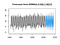Source: R Output

Now that the configuration has been selected, the forecasts can be made. With the size of the test data being 183 observations, 183 forecasts are run accordingly.

`> forecastedvalues=forecast(fitweatherarima,h=183)> forecastedvalues         Point Forecast     Lo 80     Hi 80        Lo 95     Hi 95Nov 2002       6.844297  5.209666  8.478928  4.344345165  9.344249Dec 2002       4.758306  3.069520  6.447092  2.175530989  7.341082...Dec 2017       4.794173  1.124085  8.464262 -0.818742549 10.407089Jan 2018       5.418563  1.748053  9.089073 -0.194997820 11.032123`

Using the Metrics library in R, the mean forecasts can be compared to the test set and scored on a root mean squared error (RMSE) basis.

`> library(Metrics)> rmse(forecastedvalues\$mean, test) 1.191437> mean(test) 9.559563`

The RMSE comes in at 1.91 compared to the mean of 9.55 across the test set. Given that the size of the RMSE is approximately 12% of the mean, this indicates that the model shows significant predictive power.

A Ljung-Box test is now conducted. Essentially, the test is being used to determine if the residuals of our time series follow a random pattern, or if there is a significant degree of non-randomness.

• H0: Residuals follow a random pattern
• HA: Residuals do not follow a random pattern

Note that the method for choosing a specific number of lags for Ljung-Box depends on the data in question. Given that we are working with a monthly time series, we will run the Ljung-Box test with lags 4, 8, and 12. To run this test in R, we use the following functions:

`> # Ljung-Box > Box.test(fitweatherarima\$resid, lag=4, type="Ljung-Box")Box-Ljung testdata:  fitweatherarima\$residX-squared = 6.396, df = 4, p-value = 0.1715> Box.test(fitweatherarima\$resid, lag=8, type="Ljung-Box")Box-Ljung testdata:  fitweatherarima\$residX-squared = 7.6627, df = 8, p-value = 0.4671> Box.test(fitweatherarima\$resid, lag=12, type="Ljung-Box")Box-Ljung testdata:  fitweatherarima\$residX-squared = 15.905, df = 12, p-value = 0.1956`

We see that across lags 4, 8, and 12, the null hypothesis that the lags follow a random pattern cannot be rejected and therefore our ARIMA model is free of autocorrelation.

# Part 2: Prophet Modelling

Prophet is a time series model developed by Facebook that aims to automate more technical aspects of time series forecasting, such as selection of trend and seasonality parameters.

Using the same data as above, a Prophet model is built in Python to forecast the mean temperature data for Dublin Airport, with the forecast results assessed against the test set by RMSE.

The train and test components are defined:

`train_df=dataset[:732]test_df=dataset[732:915]`

The data is formatted to make it compatible with Prophet for analysis:

`train_dataset= pd.DataFrame()train_dataset['ds'] = train_df['date']train_dataset['y']= train_df['meant']train_dataset.head(10)`

Here is a snippet of the data: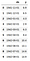Source: Jupyter Notebook Output

A standard Prophet model is defined, i.e. one where the seasonality component is being selected automatically:

`from fbprophet import ProphetProphet()prophet_basic = Prophet()prophet_basic.fit(train_dataset)`

Forecasts are made using the model:

`future= prophet_basic.make_future_dataframe(periods=183, freq='M')forecast=prophet_basic.predict(future)`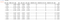Source: Jupyter Notebook Output

yhat represents the predictions that are made by the Prophet model — these will be used for the purposes of comparison with the actual test data to determine prediction accuracy.

Here are the components of the forecast: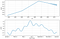Source: Jupyter Notebook Output

Prophet comes with the ability to model “changepoints”, or periods of a significant change in trend in a time series.

Let us now identify changepoints in the model.

`from fbprophet.plot import add_changepoints_to_plotfig = prophet_basic.plot(forecast)a = add_changepoints_to_plot(fig.gca(), prophet_basic, forecast)`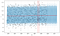Source: Jupyter Notebook Output

The identified changepoints are as follows:

`>>> prophet_basic.changepoints23    1943-10-0147    1945-10-0170    1947-09-0193    1949-08-01117   1951-08-01140   1953-07-01164   1955-07-01187   1957-06-01210   1959-05-01234   1961-05-01257   1963-04-01280   1965-03-01304   1967-03-01327   1969-02-01350   1971-01-01374   1973-01-01397   1974-12-01420   1976-11-01444   1978-11-01467   1980-10-01491   1982-10-01514   1984-09-01537   1986-08-01561   1988-08-01584   1990-07-01`

Taking these changepoints into consideration, a new set of forecasts can be developed:

`future_data = prophet_basic.make_future_dataframe(periods=183, freq = 'm')forecast_data = prophet_basic.predict(future_data)`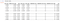Source: Jupyter Notebook Output

An updated set of forecasts is generated. The RMSE for these forecasts can now be calculated against the test set:

`>>> from sklearn.metrics import mean_squared_error>>> from math import sqrt>>> mse = mean_squared_error(test, yhat)>>> rmse = sqrt(mse)>>> print('RMSE: %f' % rmse)RMSE: 1.143002`

With an RMSE of 1.14 compared to a mean of 9.55, the Prophet model actually performed slightly better than the ARIMA model (which yielded an RMSE of 1.91).

Here is a plot of the predicted vs actual monthly temperature: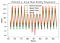Source: Jupyter Notebook Output

# Conclusion

In this example, we have seen:

• How to generate an ARIMA model in R
• Importance in accounting for seasonality trends and methods to accomplish this
• How to select the correct ARIMA modification and validate results
• Configuration of a Prophet model for time series forecasting

Many thanks for your time! The GitHub repository with associated code scripts and datasets is available here.

You can also find more of my data science content at michael-grogan.com.

Disclaimer: This article is written on an “as is” basis and without warranty. It was written with the intention of providing an overview of data science concepts, and should not be interpreted as professional advice. The findings and interpretations in this article are those of the author and are not endorsed by or affiliated with Met Éireann in any way.

## Analytics Vidhya

Analytics Vidhya is a community of Analytics and Data…

### By Analytics Vidhya

Latest news from Analytics Vidhya on our Hackathons and some of our best articles! Take a look.

By signing up, you will create a Medium account if you don’t already have one. Review our Privacy Policy for more information about our privacy practices.

Check your inbox
Medium sent you an email at to complete your subscription.

Written by

## Michael Grogan

Data Science Consultant — Expertise in time series analysis, statistics, Bayesian modeling, and machine learning with TensorFlow | michael-grogan.com

## Analytics Vidhya

Analytics Vidhya is a community of Analytics and Data Science professionals. We are building the next-gen data science ecosystem https://www.analyticsvidhya.com

Written by

## Michael Grogan

Data Science Consultant — Expertise in time series analysis, statistics, Bayesian modeling, and machine learning with TensorFlow | michael-grogan.com## Analytics Vidhya

Analytics Vidhya is a community of Analytics and Data Science professionals. We are building the next-gen data science ecosystem https://www.analyticsvidhya.com

Medium is an open platform where 170 million readers come to find insightful and dynamic thinking. Here, expert and undiscovered voices alike dive into the heart of any topic and bring new ideas to the surface. Learn more

Follow the writers, publications, and topics that matter to you, and you’ll see them on your homepage and in your inbox. Explore

If you have a story to tell, knowledge to share, or a perspective to offer — welcome home. It’s easy and free to post your thinking on any topic. Write on Medium